# Gravitational Potential Energy

## Gravitational Potential Energy

1. The expression Ug = mgh for gravitational potential energy is valid only near the surface of the earth, where one can assume that the force of gravity is constant.
2. Now we obtain the potential energy function by considering the variation of the force of gravity.
3. We know that the change in potential energy between two points is given by the negative of the work done by the conservative force:
4. UB − UA = −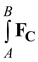.ds(11.12)
5. Since the force of gravity is central and spherically symmetric, therefore, we can express,
6.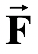c.d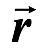= −Fr dr
7. becausec = − Fr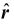and d= dr8. Therefore,UB − UA =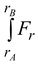dr
9. According to Newton's law of gravitation
10. Fr = GMm/r2
11. ∴UB − UA = GMm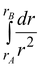12. orUB = UA + Gm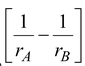13. It we assume zero level of potential energy at infinity,
14. i.e. UA = 0 as rA → ∞. Then
15. U = −GMm/r  (11.13)
16. The above equation gives the potential energy of a particle of mass m separated from the center of earth by a distance r.